Get inspired by the success stories of our students in IIT JAM MS, ISI  MStat, CMI MSc DS.  Learn More

# ISI MStat PSB 2008 Problem 2 | Definite integral as the limit of the Riemann sum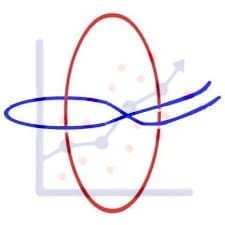This is a very beautiful sample problem from ISI MStat PSB 2008 Problem 2 based on definite integral as the limit of the Riemann sum . Let's give it a try !!

## Problem- ISI MStat PSB 2008 Problem 2

For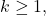let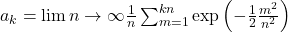Find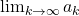.

### Prerequisites

Integration

Gamma function

Definite integral as the limit of the Riemann sum

## Solution :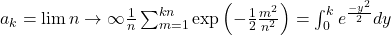, this can be written you may see in details Definite integral as the limit of the Riemann sum .

Therefore ,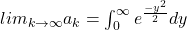----(1) , let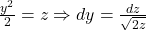Substituting we get ,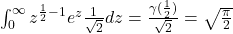Statistical Insight

Let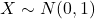i.e X is a standard normal random variable then,called folded Normal has pdf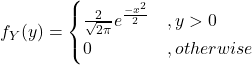. (Verify!)

So, from (1) we can say that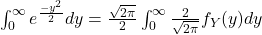( As that a PDF of folded Normal distribution ) .

## Food For Thought

Find the same when.

## Subscribe to Cheenta at Youtube

This is a very beautiful sample problem from ISI MStat PSB 2008 Problem 2 based on definite integral as the limit of the Riemann sum . Let's give it a try !!

## Problem- ISI MStat PSB 2008 Problem 2

ForletFind.

### Prerequisites

Integration

Gamma function

Definite integral as the limit of the Riemann sum

## Solution :, this can be written you may see in details Definite integral as the limit of the Riemann sum .

Therefore ,----(1) , letSubstituting we get ,Statistical Insight

Leti.e X is a standard normal random variable then,called folded Normal has pdf. (Verify!)

So, from (1) we can say that( As that a PDF of folded Normal distribution ) .

## Food For Thought

Find the same when.

## Subscribe to Cheenta at Youtube

This site uses Akismet to reduce spam. Learn how your comment data is processed.

### Knowledge Partner